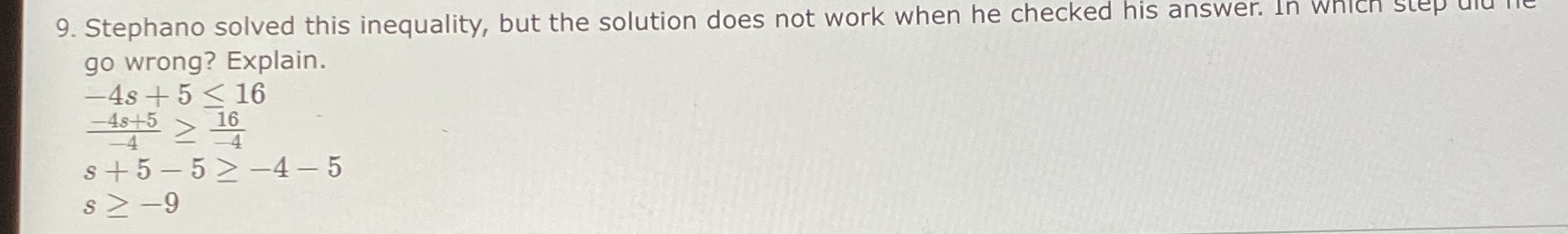### ¿Todavía tienes preguntas de matemáticas?

Pregunte a nuestros tutores expertos
Algebra
Pregunta9. Stephano solved this inequality, but the solution does not work when he checked his answer. In which step ulu ire go wrong? Explain.

$$- 4 s + 5 \leq 16$$

$$\frac { - 4 s + 5 } { - 4 } \geq \frac { 16 } { - 4 }$$

$$s + 5 - 5 \geq - 4 - 5$$

$$s \geq - 9$$

he didn't execute his division properly. $$\frac{- 4s+ 5}{- 4}\ne s+ 5$$